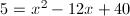# Quadratic Equations, Day 2 of 2

4 teachers like this lesson
Print Lesson

## Objective

SWBAT write quadratic equations in standard, vertex, and factored forms. SWBAT graph quadratic equations efficiently. SWBAT evaluate and solve quadratic equations.

#### Big Idea

Knowledge of quadratic equations is an important prerequisite for studying complex numbers.

## Our Goals

5 minutes

The content of this lesson is first seen in Common Core Algebra 1 and Geometry, but as we transition to the Common Core I found it was necessary to revisit it in my Algebra 2 course to ensure a foundation for our work with complex numbers.

I'll begin class again by laying out the objectives for this lesson, or series of lessons(see How long does it take?).  First, our aim is to find strategies for efficiently making a graph without technology.  Second, we aim to solve quadratic equations under any given circumstances.  Third, we will learn to transform quadratic equations into two useful forms, factored form and vertex form.

Next, we'll quickly check our progress by correcting the factoring homework from the previous lesson.  I like to do this by calling on the students one at a time to read their solutions and then asking the class if they agree or disagree with each one.  If necessary, I'll point out the usefulness of looking for common factors or of factoring out a -1.  When we're finished, I'd like to collect and grade these, but I'll allow any student to hang onto it in order to make corrections as long as it's turned in by the end of the day.

Now is the time to assign the next set of factoring practice problems, too.  They'll be due at the beginning of the next lesson.

## Strategies for Graphing Efficiently

20 minutes

A graph helps us by allowing us to see aspects of the relationship between x and y that aren't immediately obvious from an equation.  I will begin this section with a mini-lecture on graphing.  The things that students must notice about the graphs of quadratic equations are:

1. The graph is always a specific geometric shape: a parabola.
2. The graph always has either a maximum or minimum point (vertex).
3. The graph will always be symmetrical around a vertical axis running through the vertex.

Symmetry is very important because it allows us to make predictions about the overall shape of the graph based on only a few points.  I teach my students to use the following steps for making a graph efficiently:

1. Identify the y-intercept. (Use standard form, or "plug in" zero.)
2. Identify the vertex. (Use vertex form, or use x = -b/2a.)
3. Identify the roots. (Use factored form, or use the Quadratic Formula.)
4. Use symmetry to obtain one or two more point-pairs.

Really, steps 2 & 3 should sometimes be interchanged.  If the equation is easily factored, then factor it first, find the roots, and locate the vertex in between them!  In my opinion, this is an example of math practice 5 because students are being asked to use the tools at their disposal to efficiently solve the problem (MP 5).  Of course, our students will often be able to rely on technology to produce graphs for them, but these skills are important for those times when it is unavailable, as well as to help them understand what they should expect from the technology.

Now, practice, practice, practice!

20 minutes

How do you solve a problem like:?

The most important point to drive home is that you can't.  That is, you can't solve it easily in this precise form.  To use the most common solution methods, you must first set the equation equal to zero.  Once it's set equal to zero, I make sure that my students are aware of the two main solution strategies:

1. Factor the equation and use the Factor Theorem.
2. Use the Quadratic Formula (check the discriminant first!).

Next, I like to also point out the method of completing the square since I've found that some students really like to use it.  I like to point out here that for the sake of graphing, the vertex form of the equation is really the most useful because it's easy to evaluate (only one x to plug in) and it's easy to solve for a given y. (MP 7)

The point of this section is to make sure that students are able to correctly solve the equation and to correctly interpret their solutions.  In particular, they must understand that sometimes there will be no real solutions (we'll consider complex ones soon!), sometimes there will be one, but typically there will be two.  Finally, they should recognize that these solutions correspond to symmetric points on the graph.

Once again, practice!